Home MonkeyNotes Printable Notes Digital Library Study Guides Study Smart Parents Tips College Planning Test Prep Fun Zone Help / FAQ How to Cite New Title Request

REMARK: (1) Progressive and Regressive derivatives

The progressive derivative of f  at x = a is given by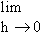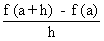, h > 0 i.e. h ® 0+ or x ® a+ and it is denoted by R f  ( a ) or by f (a + 0) or by f  ( a+) . It is also known as the Right hand derivative of f  at x = a.

The regressive derivative of  f  at x = a, is given by, h > 0 i.e. h ® 0-or x ® a- and it is denoted by Lf  (a) or by f  ( a - 0) or f " (a- ). It is also know as the Left hand derivative of  f  at x = a

It is easy to see that f  (a) exists if and only if Rf  (a) and Lf  (a) exist and are equal.Your browser does not support the IFRAME tag.

Example 1

Consider the derivability of the function f(x) = | x | at the origin.

Left hand derivative =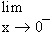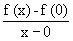=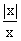=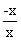=     -1

Right hand derivative =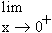===    +1

Thus f  (0 - ) ¹ f  (0 + ). Hence the function is not derivable at x = 0

Index

4. 1 Derivability At A Point
4. 2 Derivability In An Interval
4. 3 Derivability And Continuity Of A Function At A Point
4. 4 Some Counter Examples
4. 5 Interpretation Of Derivatives
4. 6 Theorems On Derivatives (differentiation Rules)
4. 7 Derivatives Of Standard Functions
4. 8 Derivative Of A Composite Function
4. 9 Differentiation Of Implicit Functions
4.10 Derivative Of An Inverse Function
4.11 Derivatives Of Inverse Trigonometric Functions
4.12 Derivatives Of Exponential & Logarithmic Functions
4.13 Logarithmic Differentiation
4.14 Derivatives Of Functions In Parametric Form
4.15 Higher order Derivatives

Chapter 5All Contents Copyright © All rights reserved.
Further Distribution Is Strictly Prohibited.

1209814 PinkMonkey users are on the site and studying right now.